# ISPMT Function

Calculates the interest paid during a specific period of Investment

## What is the ISPMT Function?

The ISPMT Function is categorized under Excel Financial functions. The function will calculate the interest paid during a specific period of Investment.

ISPMT is useful to a financial analyst as it helps predict interest payments while preparing revenue forecasts, annual budgets, etc. For example, suppose a business takes out a loan and we need to know how much interest would be paid in a particular period. Using the ISPMT function, we can find out the interest due in the future.

### Formula

=ISPMT(rate,per,nper,pv)

The ISPMT function uses the following arguments:

1. Rate (required argument) – This is the interest rate on the investment or loan.
2. Per (required argument) – This is the period for which we want to calculate the interest. It must be an integer between 1 and nper.
3. Nper (required argument) – The number of periods over which the loan or investment must be paid back.
4. Pv (required argument) – This is the present value of the loan/investment. It can be the total value of payments made to date.

Remember that outgoing payments are represented by negative numbers and incoming payments are represented by positive numbers.

For example, the cash paid out, such as interest on a loan, is represented by a negative number and the cash received, such as a dividend check on investments made, is represented by a positive number.

### How to use the ISPMT Function in Excel?

To understand the uses of the ISPMT function, let’s consider a few examples:

#### Example 1

Suppose a business takes out a \$5 million loan. It needs to be repaid in 10 years and the interest is charged at 5% per year. The interest needs to be paid on a monthly basis.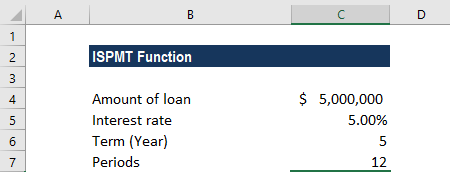The payments are made monthly, so we will need to convert the annual interest rate of 5% into the monthly rate (=5%/12) and the number of periods from years to months (=5*12).

The modified data will look like this: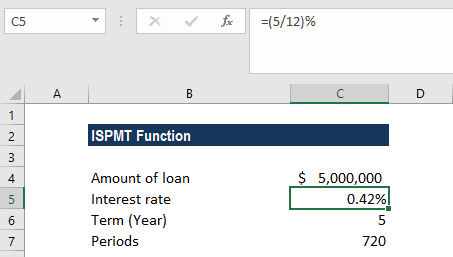The interest is divided by 12 to get the monthly rate. The periods are multiplied by 5 as its monthly payments. The formula for the interest paid is: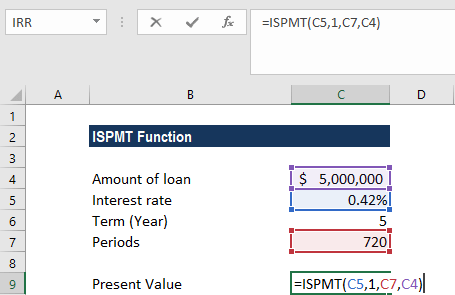We get the result below: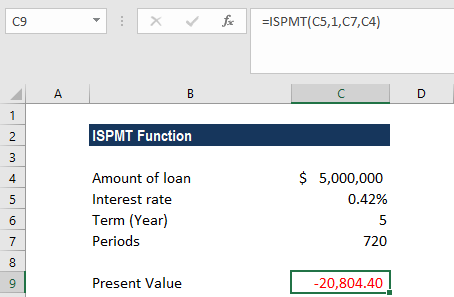The value is negative as it represents outgoing payments.

#### Example 2

Suppose we need to calculate the interest payment for the 25th week. The data is given below: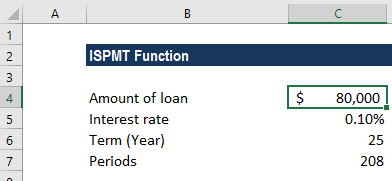As the payments are done on a weekly basis, we converted the interest rate of 5% to 52 weeks, so it is 0.10%. The loan is for four years, so the number of weeks is 208 (52×4). For the interest payment, the formula used is: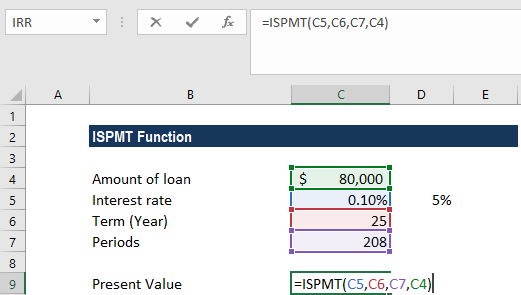We get the result below: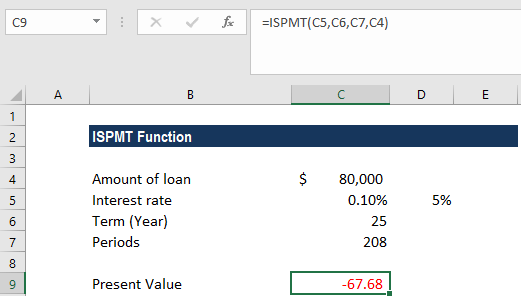### A few notes about the ISPMT Function

1. In the ISPMT function, remember that while calculating monthly or quarterly payments, the interest rate or the number of periods need to be converted to months or quarters.

To solve the problem, we need to ensure that the rate and the nper arguments are expressed in the correct units, i.e.:

• Months = 12 * years
• Quarters = 4 * years
• Weeks = 52 * years
• Monthly rate = Annual rate / 12
• Quarterly rate = Annual rate / 4
• Weekly rate = Annual rate / 52
1. #VALUE! error – Occurs when any of the given arguments is non-numeric.
2. The payment returned by ISPMT includes the principal and interest but will not include any taxes, reserve payments, or fees.
3. The ISPMT function was introduced in MS Excel 2000.

Thanks for reading CFI’s guide to important Excel functions! By taking the time to learn and master these functions, you’ll significantly speed up your financial analysis. To learn more, check out these additional CFI resources:

• Excel Functions for Finance